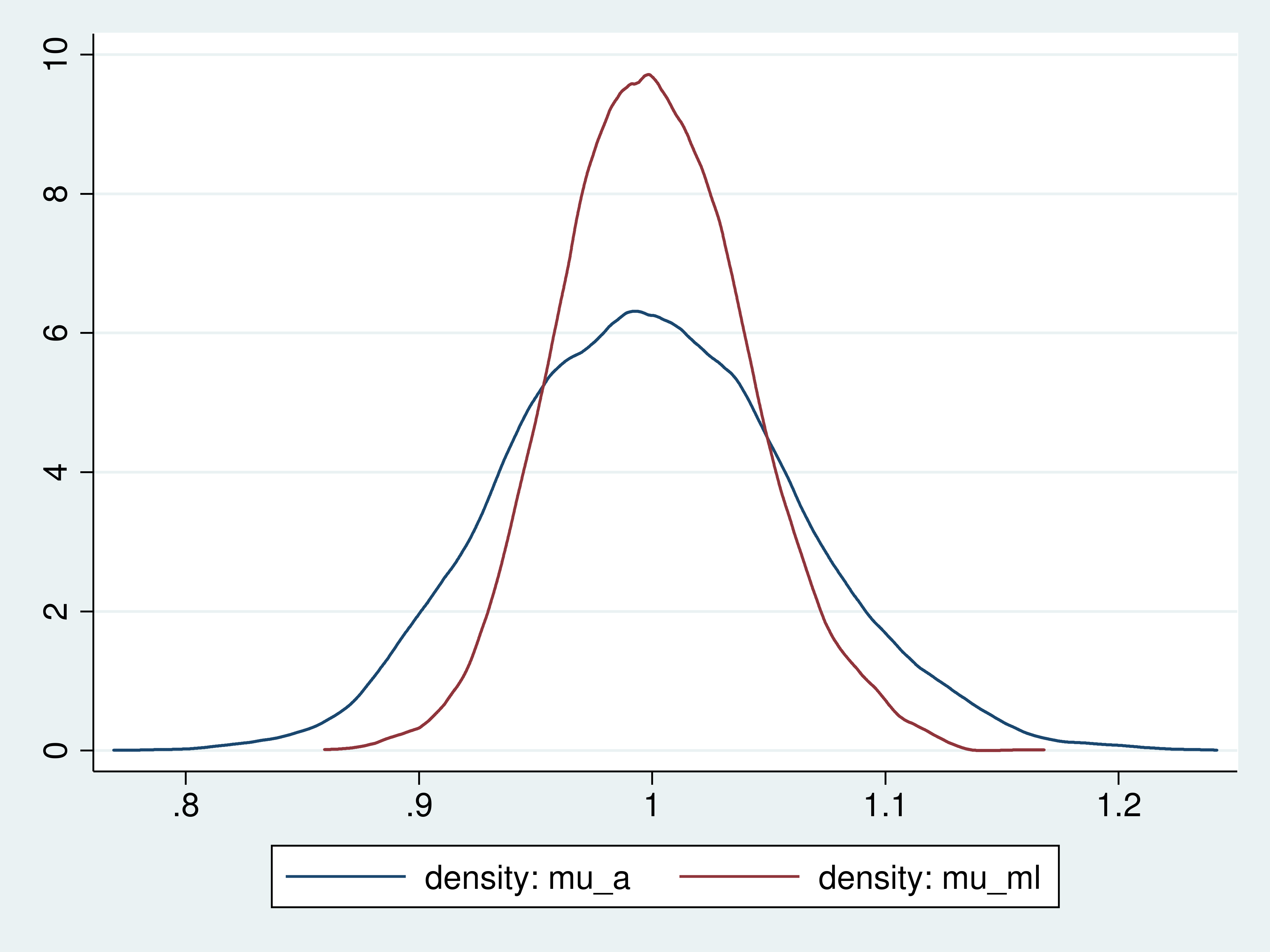Home > Statistics > Efficiency comparisons by Monte Carlo simulation

## Efficiency comparisons by Monte Carlo simulation

Overview

In this post, I show how to use Monte Carlo simulations to compare the efficiency of different estimators. I also illustrate what we mean by efficiency when discussing statistical estimators.

I wrote this post to continue a dialog with my friend who doubted the usefulness of the sample average as an estimator for the mean when the data-generating process (DGP) is a $$\chi^2$$ distribution with $$1$$ degree of freedom, denoted by a $$\chi^2(1)$$ distribution. The sample average is a fine estimator, even though it is not the most efficient estimator for the mean. (Some researchers prefer to estimate the median instead of the mean for DGPs that generate outliers. I will address the trade-offs between these parameters in a future post. For now, I want to stick to estimating the mean.)

In this post, I also want to illustrate that Monte Carlo simulations can help explain abstract statistical concepts. I show how to use a Monte Carlo simulation to illustrate the meaning of an abstract statistical concept. (If you are new to Monte Carlo simulations in Stata, you might want to see Monte Carlo simulations using Stata.)

Consistent estimator A is said to be more asymptotically efficient than consistent estimator B if A has a smaller asymptotic variance than B; see Wooldridge (2010, sec. 14.4.2) for an especially useful discussion. Theoretical comparisons can sometimes ascertain that A is more efficient than B, but the magnitude of the difference is rarely identified. Comparisons of Monte Carlo simulation estimates of the variances of estimators A and B give both sign and magnitude for specific DGPs and sample sizes.

The sample average versus maximum likelihood

Many books discuss the conditions under which the maximum likelihood (ML) estimator is the efficient estimator relative to other estimators; see Wooldridge (2010, sec. 14.4.2) for an accessible introduction to the modern approach. Here I compare the ML estimator with the sample average for the mean when the DGP is a $$\chi^2(1)$$ distribution.

Example 1 below contains the commands I used. For an introduction to Monte Carlo simulations see Monte Carlo simulations using Stata, and for an introduction to using mlexp to estimate the parameter of a $$\chi^2$$ distribution see Maximum likelihood estimation by mlexp: A chi-squared example. In short, the commands do the following $$5,000$$ times:

1. Draw a sample of 500 observations from a $$\chi^2(1)$$ distribution.
2. Estimate the mean of each sample by the sample average, and store this estimate in m_a in the dataset efcomp.dta.
3. Estimate the mean of each sample by ML, and store this estimate in m_ml in the dataset efcomp.dta.

Example 1: The distributions of the sample average and the ML estimators

. clear all
. set seed 12345
. postfile sim  mu_a mu_ml using efcomp, replace
. forvalues i = 1/5000 {
2.     quietly drop _all
3.     quietly set obs 500
4.     quietly generate double y = rchi2(1)
5.     quietly mean y
6.     local mu_a         =  _b[y]
7.     quietly mlexp (ln(chi2den({d=1},y)))
8.     local mu_ml   =  _b[d:_cons]
9.     post sim (mu_a') (mu_ml')
10. }
. postclose sim
. use efcomp, clear
. summarize

Variable |        Obs        Mean    Std. Dev.       Min        Max
-------------+---------------------------------------------------------
mu_a |      5,000    .9989277    .0620524   .7792076   1.232033
mu_ml |      5,000    1.000988    .0401992   .8660786   1.161492


The mean of the $$5,000$$ sample average estimates and the mean of the $$5,000$$ ML estimates are each close to the true value of $$1.0$$. The standard deviation of the $$5,000$$ sample average estimates is $$0.062$$, and it approximates the standard deviation of the sampling distribution of the sample average for this DGP and sample size. Similarly, the standard deviation of the $$5,000$$ ML estimates is $$0.040$$, and it approximates the standard deviation of the sampling distribution of the ML estimator for this DGP and sample size.

We conclude that the ML estimator has a lower variance than the sample average for this DGP and this sample size, because $$0.040$$ is smaller than $$0.062$$.

To get a picture of this difference, we plot the density of the sample average and the density of the ML estimator. (Each of these densities is estimated from $$5,000$$ observations, but estimation error can be ignored because more data would not change the key results.)

Example 2: Plotting the densities of the estimators

. kdensity mu_a,   n(5000) generate(x_a   den_a)   nograph

. kdensity mu_ml,  n(5000) generate(x_ml  den_ml)  nograph

. twoway (line den_a x_a) (line den_ml x_ml)


Densities of the sample average and ML estimatorsThe plots show that the ML estimator is more tightly distributed around the true value than the sample average.

That the ML estimator is more tightly distributed around the true value than the sample average is what it means for one consistent estimator to be more efficient than another.

Done and undone

I used Monte Carlo simulation to illustrate what it means for one estimator to be more efficient than another. In particular, we saw that the ML estimator is more efficient than the sample average for the mean of a $$\chi^2(1)$$ distribution.

Many other estimators fall between these two estimators in an efficiency ranking. Generalized method of moments estimators and some quasi-maximum likelihood estimators come to mind and might be worth adding to these simulations.

Reference

Wooldridge, J. M. 2010. Econometric Analysis of Cross Section and Panel Data. 2nd ed. Cambridge, Massachusetts: MIT Press.

Categories: Statistics Tags: